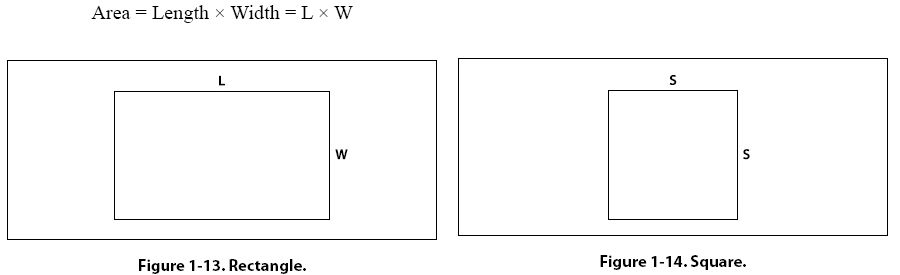Order of Operation for Algebraic Equations 1. Parentheses 2. Exponents 3. Multiplication and Division 4. Addition and Subtraction Use the acronym PEMDAS to remember the order of operation in algebra. PEMDAS is an acronym for parentheses, exponents, multiplication, division, addition, and subtraction. To remember it, many use the sentence, “Please Excuse My Dear Aunt Sally." Always remember, however, to multiply/divide or add/subtract in one sweep from left to right, not separately. Computing Area of Two-dimensional Solids Area is a measurement of the amount of surface of an object. Area is usually expressed in such units as square inches or square centimeters for small surfaces or in square feet or square meters for larger surfaces. Rectangle A rectangle is a four-sided figure with opposite sides of equal length and parallel. [Figure 1-13] All of the angles are right angles. A right angle is a 90° angle. The rectangle is a very familiar shape in mechanics. The formula for the area of a rectangle is: Area = Length x Width = L x W Example: An aircraft floor panel is in the form of a rectangle having a length of 24 inches and a width of 12 inches. What is the area of the panel expressed in square inches? First, determine the known values and substitute them in the formula. A = L x W = 24 inches x 12 inches = 288 square inches Square A square is a four-sided figure with all sides of equal length and parallel. [Figure 1-14] All angles are right angles. The formula for the area of a square is: Area = Length x Width = L x WSince the length and the width of a square are the same value, the formula for the area of a square can also be written as: Area = Side x Side = S2 Example: What is the area of a square access plate whose side measures 25 inches? First, determine the known value and substitute it in the formula. A = L x W = 25 inches x 25 inches = 625 square inches
 ©AvStop Online Magazine                                                                                                                                                      Contact Us              Return To Books